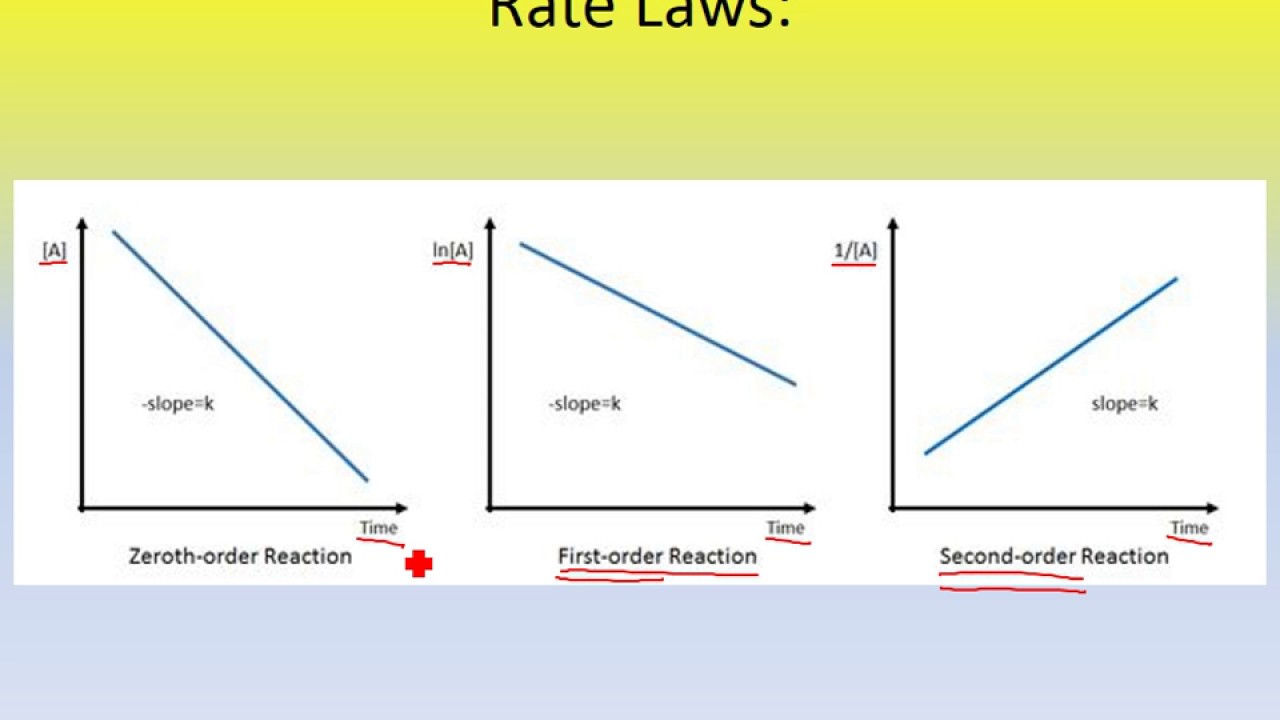Page 1 of 1

### rate laws and graphs

Posted: Tue Mar 12, 2019 11:46 pm
Is it important that we understand/are able to implement graphs of rate laws and integrated rate laws for solving problems?

### Re: rate laws and graphs

Posted: Tue Mar 12, 2019 11:58 pm
I think it is important to understand the graphs. I doubt we would need to draw them, but knowing the what the slopes are for different orders is probably useful. I think some practice problems even incorporate the graphs, and it might be hard to solve them if you do not know what the axes/slope would be if they aren’t labeled.

### Re: rate laws and graphs

Posted: Wed Mar 13, 2019 1:09 pm
It is important to know what the integrated rate law for a 0, 1st, and 2nd order looks like when graphed, as well as what would cause them to be a straight line.

### Re: rate laws and graphs

Posted: Wed Mar 13, 2019 10:12 pm
You should probably know what the graphs generally look like and how the graphs are related to the equations.

### Re: rate laws and graphs

Posted: Mon Mar 09, 2020 1:24 pm
For your reference, here are the graphs showing the slope line trends and axes labels for each order of reaction: